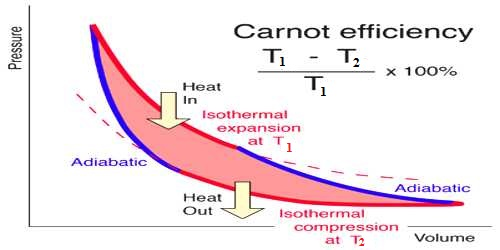# Efficiency of Carnot’s Engine

Efficiency of Carnot’s Engine

The Carnot’s engine is called an ideal engine. The most efficient heat engine cycle is the Carnot cycle, consisting of two isothermal processes and two adiabatic processes. The ratio of the amount of heat converted into work and the amount of heat absorbed from the source in each cycle by this engine is called the efficiency of the engine. Its efficiency is more than any practical engine.

Suppose heat taken by the working substance (gas) of this engine is Q1 and heat rejected is Q2. The amount of heat transformed into work = Q1 – Q2

Efficiency of the engine, η = [Heat transformed into work / Heat absorbed from the source]

= (Q1 – Q2) / Q1 = 1 – (Q2 / Q2)

In order to express the efficiency of a Carnot’s cycle in terms of temperature, the transformation of (Q2 / Q2) to (T2/T1) is needed. In that case, efficiency of the engine, η = 1 – (T2/T1)

When expressed in terms of percentage, η = [(T1 – T2) / T1] x 100%

Realization: What are the ideas you can make about the engine from the efficiency of the engine?

• From the efficiency of the engine, it is observed that it depends only on the temperatures of the heat source and heat sink T1 and T2, respectively but not on the nature of working substance.
• The efficiency of all the reversible engines working between two fixed temperatures is equal.
• Since T1 > (T1 – T2), hence the efficiency of an engine can never be 100%.
• As the difference in temperatures of the heat source and heat sink increases the efficiency of the engine also increases.